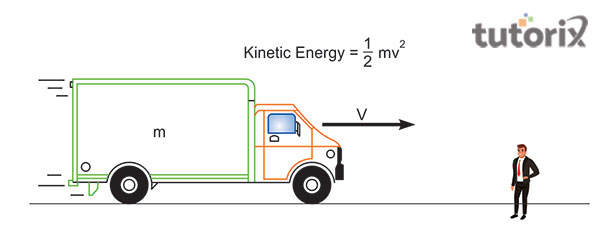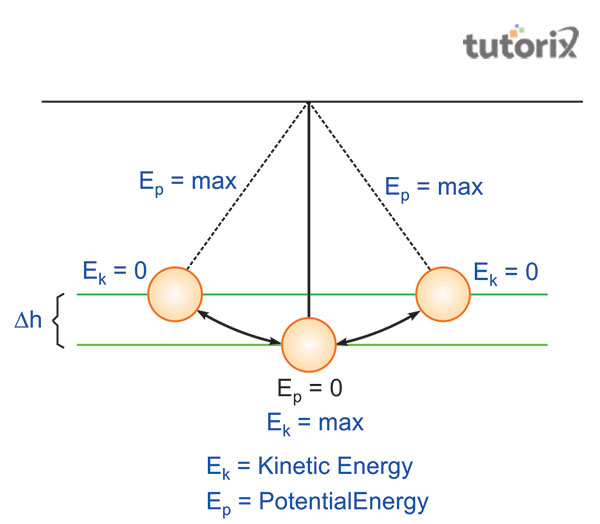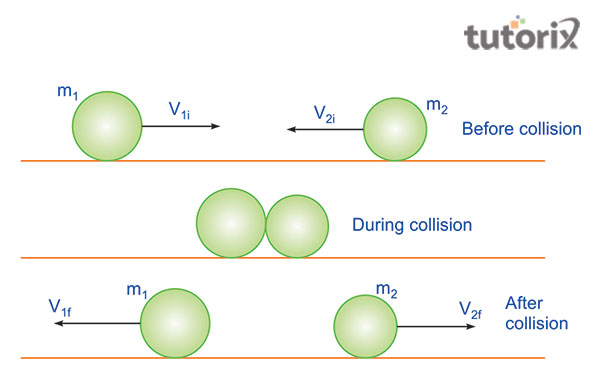# Relation Between Kinetic Energy and Momentum

## Introduction

Both the aspects of the momentum and kinetic energy are crucial in the field of physics, as momentum is said to describe the relationship with certain specified aspects. These aspects are speed, mass and direction for a concerned object having considerable mass. On the other hand, kinetic energy is the energy within a mass associated with motion. The energy within the body is inherited due to the motion exerted on a body. Kinetic energy is stated as the potentiality to conduct work.

## Definition of Kinetic energy

In the subject of physics, several types of energies are noticed that are conserved within a body. However, the energy that supports the body to conduct any motion is defined as the kinetic energy. When the body is said to be accelerated with motion, then it is understood that a certain significant force is applied to the body (Li et al. 2019).

However, one needs to understand that certain work needs to be done when the force is applied. This work is transformed into the form of energy that is supporting the body to conduct movement with a constant velocity.

This transference of energy is defined as kinetic energy (Jeong et al. 2020). Simple actions conducted by us in our day-to-day life such as walking, throwing, and jumping and so on include kinetic energy.Figure 1: Concept of Kinetic Energy

## Units and examples of kinetic energy

Kinetic energy is said to have been a scalar quantity and is only used to describe the aspect of magnitude. There are two units of Kinetic energy, in (SI unit) or International System of Units is expressed as, 1 kg.m2.s-2, and the unit is known as, Joule or (J) (Kuya et al. 2018). The other unit, in (CGS) or centimeter-gram-seconds is erg.

Some examples of kinetic energy are listed below.

Such as, a truck going down a road tends to have more kinetic energy than a pool car travelling at the same speed. This is because the mass of the truck is more than the pool car. It can also be said as an example of kinetic energy when the river flows across its lane as water seems to possess a certain mass and velocity.

## The formula for Kinetic energy

The formula for kinetic energy for a particular body is expressed as, KE = 1/2 m * v2, where, KE is the kinetic energy possessed by a body, v is the velocity of the concerned object of mass and finally m stand for the mass of the body (Physicsclassroom.com, 2022). The formula of kinetic energy can be easily derived from the basic formula associated with the work, where work done is defined by force exerted on the body along a distance denoted by d.

## Types of kinetic energy

Several types of kinetic energy are noticed that amount to five in number and that includes, including radiant energy, mechanical energy, thermal energy, electrical energy, and sound energy (Ducksters.com, 2022). Examples of radiant energies relate to the light ultraviolet rays of gamma, and the beating of drums can be considered as examples of sound energy.

## What is potential energy?

Potential energy is defined as the energy that is inherited by a body when it is in its position in the state. This kind of energy is said to be stored within the body when the body is in the position of rest. However, three types of energies are found here, that include, potential electrical, chemical energy and lastly, nuclear energy.Figure 2: Concept of Potential Energy

## Examples of potential energy

Several examples are found in order to determine potential energy, for example, the sitting of a rocket on the peripheral side of a cliff. The food that we intake is referred to as the chemical potential energy, that provides us with energy to perform work.

## Definition of momentum and its formula

Momentum in the field of physics is defined as the mass that has motion within it, however, the amount of momentum has two variables (Livescience.com, 2022). These two variables include what amount of mass is moving and at what speed (Ducksters.com, 2022). Therefore, the formula of potential energy is expressed as, m * g * h, where, ''m  denotes'' mass ,''g  denotes ''gravitational energy and finally, ''h  denotes'' height  respectively.Figure 3: Concept of Momentum

## Examples of momentum

Certain examples of momentum are listed below, for example, the moving of a heavy truck, the flying of a baseball through the air, the firing of a bullet from a gun, and a train moving with a speed of 120 km/h and so on.

## Relation between kinetic energy and momentum

Both the notions of kinetic energy and momentum in physics are intricately related. On one hand, kinetic energy is the energy possessed by a body when it gains energy and on the other, momentum is said when the mass of an object is in motion.

Mathematically, it can be stated as, KE = 1/2 m * v and p = m * v, therefore, equating both, KE = 1/2 m * v * v, this equals to KE = 1/2 p * v.

## Conclusion

However, in this tutorial, an in-depth idea has been delivered by stating the intricate relationship between the kinetic energy and momentum. It can be stated, that the body possesses the kinetic energy, whereas, momentum refers to a mass of a body associative with motion.

## FAQs

Q1. What is the formula of work done?

Ans. The formula for work done in the field of physics can be expressed as W = F * d, where, F is force and d is the distance of the object.

Q2. What is the example of potential energy?

Ans. An example of potential energy is gravitational force as it can be determined by the formula mgh.

Q3. What is the SI unit of kinetic energy?

Ans. The SI unit of kinetic energy can be expressed as, Joules (J) that is equal to kgm2s-2.